# 一、SVM简介

## （一）Support Vector Machine

1. 支持向量机（SVM：Support Vector Machine）是机器学习中常见的一种分类算法。
2. 线性分类器，也可以叫做感知机，其中表示的是一种算法。
3. 在实际应用中，我们往往遇到这样的问题：

给定一些数据点，它们分别属于两个不同的类。我们现在要找到一个线性分类器把这些数据分成AB两类。最简单的办法当然是，画一条线，然后将它们分成两类。线的一侧，属于A类，另一侧，则属于B类。SVM算法可以让我们找到这样一个最佳的线（超平面），来划分数据。相比于KNN之类的算法，SVM算法只需要计算一次，得出最佳线（超平面）即可。面对测试数据，只需要判断数据点落在线的哪一侧，就可以知道该数据点所属分类了。比起KNN每次都需要计算一遍邻居点的分类，SVM算法显得简单无比。

## （二）Sklearn参数详解—SVM

1 sklearn.svm.LinearSVC(penalty='l2', loss='squared_hinge', dual=True, tol=0.0001, C=1.0, multi_class='ovr', fit_intercept=True, intercept_scaling=1, class_weight=None, verbose=0, random_state=None, max_iter=1000)
• penalty:正则化参数，L1和L2两种参数可选，仅LinearSVC有。
• loss:损失函数，有‘hinge’和‘squared_hinge’两种可选，前者又称L1损失，后者称为L2损失，默认是是’squared_hinge’，其中hinge是SVM的标准损失，squared_hinge是hinge的平方。
• dual:是否转化为对偶问题求解，默认是True。
• tol:残差收敛条件，默认是0.0001，与LR中的一致。
• C:惩罚系数，用来控制损失函数的惩罚系数，类似于LR中的正则化系数。
• multi_class:负责多分类问题中分类策略制定，有‘ovr’和‘crammer_singer’ 两种参数值可选，默认值是’ovr’，'ovr'的分类原则是将待分类中的某一类当作正类，其他全部归为负类，通过这样求取得到每个类别作为正类时的正确率，取正确率最高的那个类别为正类；‘crammer_singer’ 是直接针对目标函数设置多个参数值，最后进行优化，得到不同类别的参数值大小。
• fit_intercept:是否计算截距，与LR模型中的意思一致。
• class_weight:与其他模型中参数含义一样，也是用来处理不平衡样本数据的，可以直接以字典的形式指定不同类别的权重，也可以使用balanced参数值。
• verbose:是否冗余，默认是False. random_state:随机种子的大小。
• max_iter:最大迭代次数，默认是1000。

对象

• coef_:各特征的系数（重要性）。
• intercept_:截距的大小（常数值）。
1 sklearn.svm.NuSVC(nu=0.5, kernel='rbf', degree=3, gamma='auto', coef0=0.0, shrinking=True, probability=False, tol=0.001, cache_size=200, class_weight=None, verbose=False, max_iter=-1, decision_function_shape='ovr', random_state=None))
• nu:训练误差部分的上限和支持向量部分的下限，取值在（0，1）之间，默认是0.5
• kernel:核函数，核函数是用来将非线性问题转化为线性问题的一种方法，默认是“rbf”核函数，常用的核函数有以下几种：

linear

poly

rbf

sigmod

sigmod核函数

precomputed

• degree:当核函数是多项式核函数的时候，用来控制函数的最高次数。（多项式核函数是将低维的输入空间映射到高维的特征空间）
• gamma:核函数系数，默认是“auto”，即特征维度的倒数。
• coef0:核函数常数值(y=kx+b中的b值)，只有‘poly’和‘sigmoid’核函数有，默认值是0。
• max_iter:最大迭代次数，默认值是-1，即没有限制。
• probability:是否使用概率估计，默认是False。
• decision_function_shape:与'multi_class'参数含义类似。
• cache_size:缓冲大小，用来限制计算量大小，默认是200M。

对象

• support_:以数组的形式返回支持向量的索引。
• support_vectors_:返回支持向量。
• n_support_:每个类别支持向量的个数。
• dual_coef_:支持向量系数。
• coef_:每个特征系数（重要性），只有核函数是LinearSVC的时候可用。
• intercept_:截距值（常数值）。
1 sklearn.svm.SVC(C=1.0, kernel='rbf', degree=3, gamma='auto', coef0=0.0, shrinking=True, probability=False, tol=0.001, cache_size=200, class_weight=None, verbose=False, max_iter=-1, decision_function_shape='ovr', random_state=None)
• C:惩罚系数。

SVC和NuSVC方法基本一致，唯一区别就是损失函数的度量方式不同（NuSVC中的nu参数和SVC中的C参数）
• decision_function(X):获取数据集X到分离超平面的距离。

• fit(X, y):在数据集(X,y)上使用SVM模型。
• get_params([deep]):获取模型的参数。
•  predict(X):预测数据值X的标签。
• score(X,y):返回给定测试集和对应标签的平均准确率。

# 二、感知机简介

感知机(preceptron)是线性分类的二分类模型，输入为实例的特征向量，输出为实例的类别，分别用 1 和 -1 表示。感知机将输入空间(特征空间)中的实例划分为正负两类分离的超平面，旨在求出将训练集进行线性划分的超平面，为此，导入基于误分类的损失函数，利用梯度下降法对损失函数进行极小化，求得最优解。感知机是神经网络和支持向量机的基础。

# 三、超平面

1. 当我们的数据点集是二维的时候（意味着有两个特征），我们只需要用一根线，就可以将数据分成两个部分。
2. 当我们的数据点集是三维的时候（意味着有三个特征），我们需要一个二维的平面，才能把数据分成两个部分。
3. 当我们的数据点集为N维的时候（意味着有N个特征），此时我们需要一个N-1维的超平面，才可以把数据分成两个部分。
4. 我们有无数个超平面可以将数据集分成两个部分,我们应该怎么找到最佳的分割线呢？https://www.kesci.com/home/project/5cf08e4dc84534002b03cdfd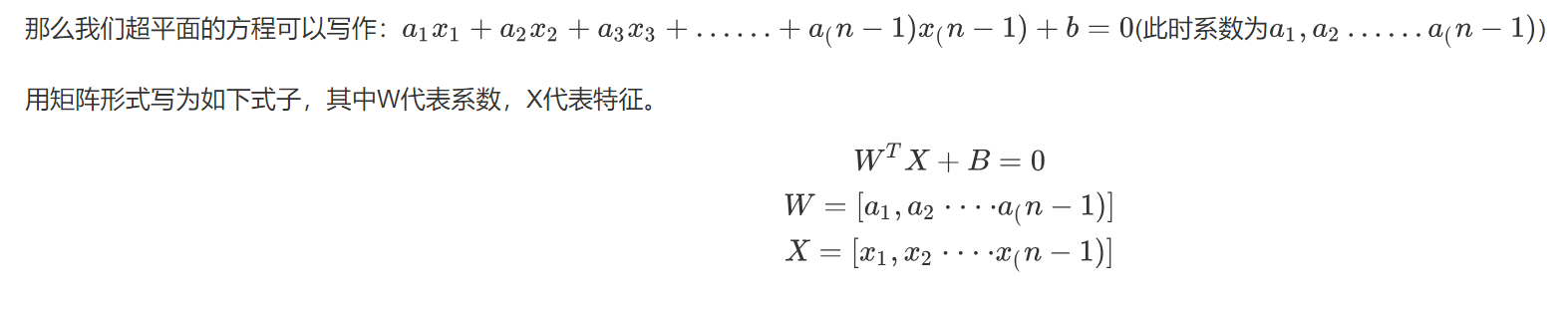• 虚线中心的绿色实线，就是我们在保持超平面方向不变的时候，得到的最佳超平面。

• 两条虚线之间的垂直距离，代表这这个超平面的分类间隔。

• 改变超平面的方向的时候，我们会得到不同方向的最佳超平面。

• “分类间隔”最大的那个超平面，就是真正的最佳超平面。即最佳超平面为两条虚线的中轴线
• 该平面所对应的两侧虚线穿过的样本点，就是SVM中的支持样本点，被称为“支持向量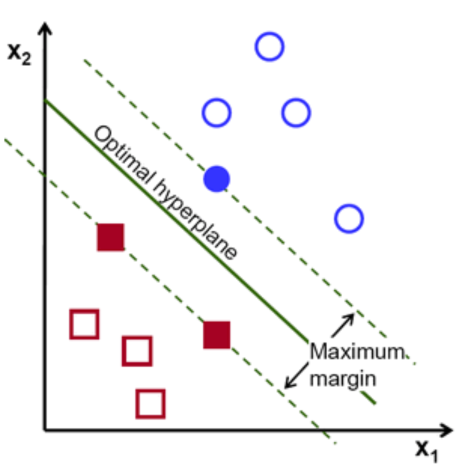# 四、核函数

## （一）核函数介绍

https://blog.csdn.net/batuwuhanpei/article/details/52354822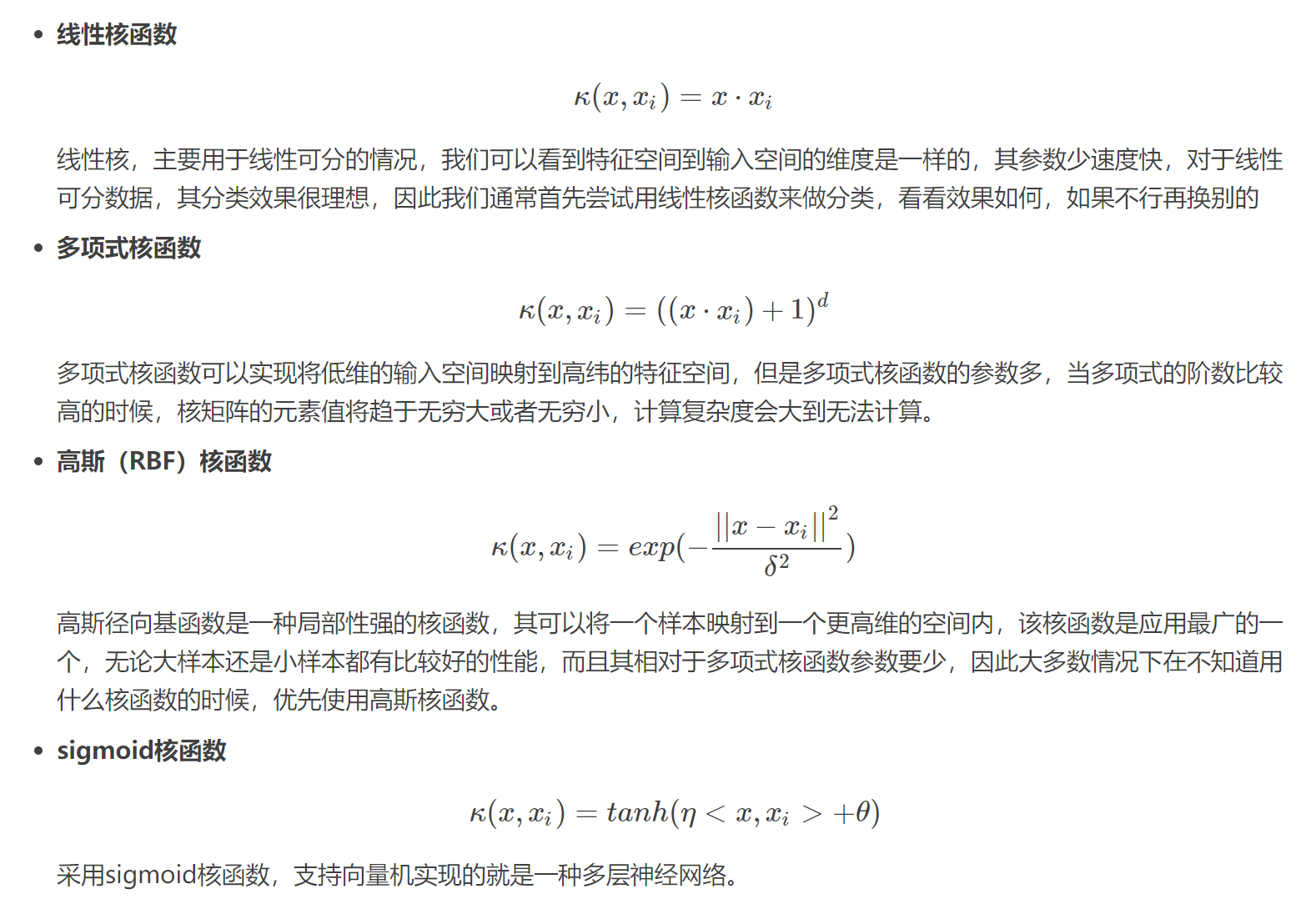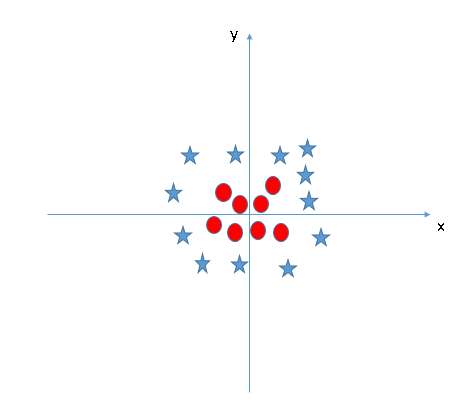理论上任意的数据样本都能够找到一个合适的映射，使得这些在低维空间不能划分的样本到高维空间中之后能够线性可分。以二维平面的数据为例，我们可以找到一个映射，将二维平面的点映射到三维平面之中。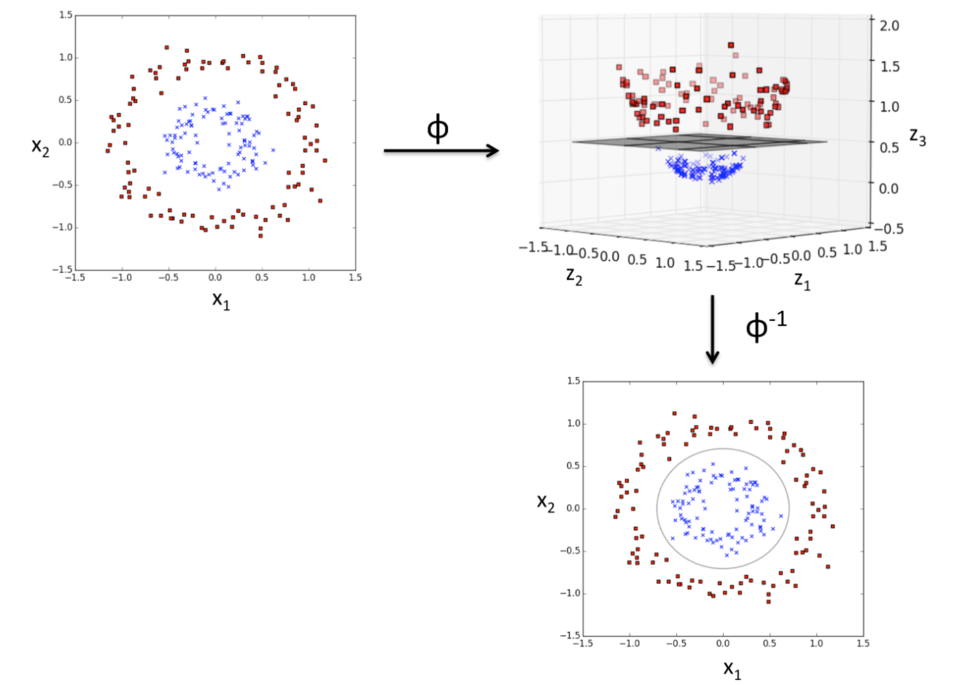• Linear Kernel 线性核函数
• Polynomial Kernel 多项式核函数
• Radial Basis Function (RBF) kernel 径向基核函数（RBF） …………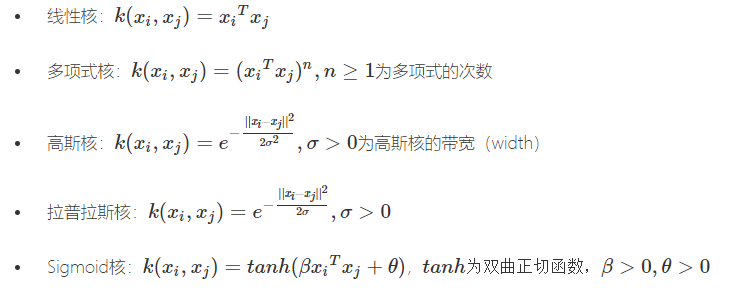## （二）核函数的选择

在选用核函数的时候，希望通过将输入空间内线性不可分的数据映射到一个高纬的特征空间内使得数据在特征空间内是可分的，如果我们对我们的数据有一定的先验知识，就利用先验来选择符合数据分布的核函数；如果不知道的话，通常使用交叉验证的方法，来试用不同的核函数，误差最下的即为效果最好的核函数，或者也可以将多个核函数结合起来，形成混合核函数。在吴恩达的课上，也曾经给出过一系列的选择核函数的方法：

• 如果特征的数量大到和样本数量差不多，则选用LR或者线性核的SVM；
• 如果特征的数量小，样本的数量正常，则选用SVM+高斯核函数；
• 如果特征的数量小，而样本的数量很大，则需要手工添加一些特征从而变成第一种情况。

# 五、乳腺癌数据集（选取2特征）实验

 1 import numpy as np
2 import matplotlib.pyplot as plt
3 from sklearn import svm
4 from sklearn.datasets import make_blobs,load_breast_cancer
5
7 # dict_keys(['data', 'target', 'target_names', 'DESCR', 'feature_names', 'filename'])
8 data1 = load_breast_cancer().data
9 target1 = load_breast_cancer().target
10
11 print('data1,target1',data1.shape,target1.shape)
12 # data1,target1 (569, 30) (569,)
13 #一共有三十列特征，可以任意选择特征的数目,这里以二维为例
14 data = load_breast_cancer().data[:, 0:2]
15 target = load_breast_cancer().target
16 print(np.unique(target))
17
18 #创建SVM模型
19 #选择核函数为'linear'
20 #C: 目标函数的惩罚系数C，用来平衡分类间隔margin和错分样本的，default C = 1.0；
21 #kernel：参数选择有RBF, Linear, Poly, Sigmoid, 默认的是"RBF"
22
23 model = svm.SVC(kernel='linear',C=10000)
24
25 #使用模型训练数据集
26 model.fit(data, target)
27
28 #绘制数据集散点图
29 # plt.scatter(data[:, 0], data[:, 1], c=target)
30 plt.scatter(data[:, 0], data[:, 1], c=target, s=30, cmap=plt.cm.prism)
31
32 #创建坐标轴刻度线
33 axis = plt.gca()
34 x_limit = axis.get_xlim()
35 y_limit = axis.get_ylim()
36
37 x = np.linspace(x_limit, x_limit, 50)
38 y = np.linspace(y_limit, y_limit, 50)
39 print('x,y',x.shape,y.shape)#x,y (50,) (50,)
40 X, Y = np.meshgrid(x, y)
41 print('X, Y ',X.shape, Y.shape )#X, Y  (50, 50) (50, 50)
42 xy = np.c_[X.ravel(), Y.ravel()]
43 print('xy',xy.shape)#xy (2500, 2)
44
45
46 # 创建最佳分割线
47 decision_line = model.decision_function(xy).reshape(Y.shape) #计算样本点到分割超平面的函数距离
48 49 # 绘制分割线的图 50 axis.contour(X, Y, decision_line, colors = 'k', levels=, 51 linestyles=['-']) 52 53 # 展示一下支持向量点 54 plt.scatter(model.support_vectors_[:, 0], model.support_vectors_[:, 1], s=100, 55 linewidth=1, facecolors='none', edgecolors='k') 56 plt.show()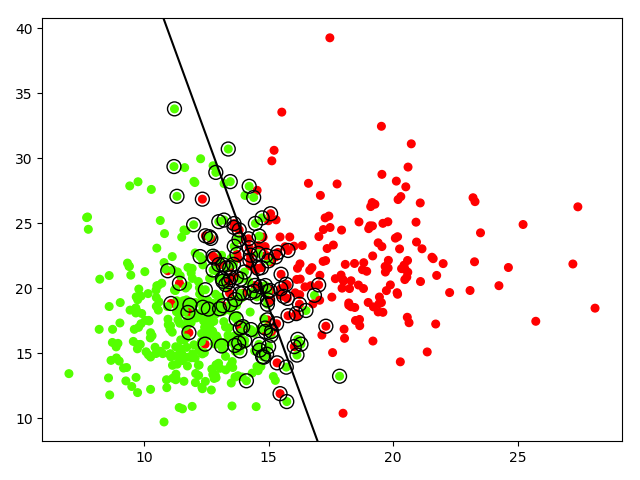## （一）线性完全可分问题 hard margin classfication

https://www.jianshu.com/p/6ebdfe0cf1a4

 1 # coding = utf-8
2 import numpy as np
3 import matplotlib.pyplot as plt
4 from sklearn import svm
5 from sklearn.datasets import make_blobs
6
7 a,b = make_blobs()
8 print('make_blobs()',a.shape,b.shape)#make_blobs() (100, 2) (100,)
9
10 x,y = make_blobs(n_samples=40,centers=2,random_state=9)
11 print('x,y',x.shape,y.shape)#x,y (40, 2) (40,)
12
13 '''
14 centers就是数据分布点，我们现在解决的是二分类问题，\
15 那么就需要两个center，数据点都分别围绕着center进行分布
16 random_state确定一个数字后，每次运行都会出现相同的随机数。
17 '''
18 plt.scatter(x[:,0],x[:,1],c = y,cmap=plt.cm.Paired)
19 '''
20 plt.scatter中的c代表color，cmap代表colormap，可选
21 s是size, c=y是标签分类和plt.cm.Paired联合使用
22 '''
23 plt.show()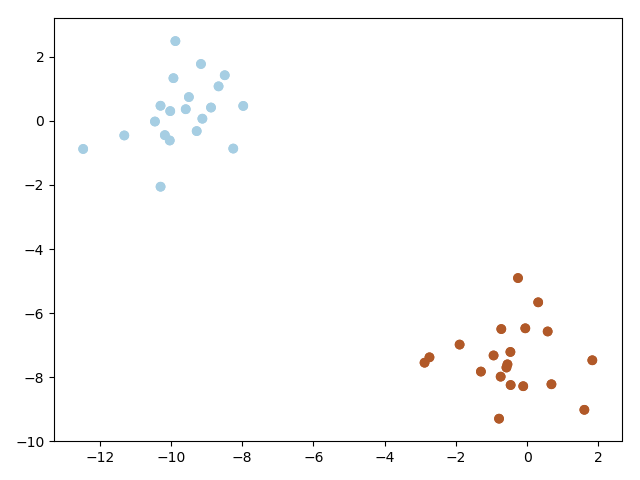## （二）线性不可分SVM

 1 import numpy as np
2 import matplotlib.pyplot as plt
3 from sklearn import svm
4 import warnings
5 warnings.filterwarnings('ignore')
6 np.random.seed(0)
7 X=np.random.randn(300,2)
8 Y=np.logical_xor(X[:,0]>0, X[:,1]>0)
9 xx,yy = np.meshgrid(np.linspace(-3,3,500),np.linspace(-3,3,500))
10 #fit
11 clf = svm.SVC() #所有参数采用默认
12 clf.fit(X,Y)
13 xy = np.vstack([xx.ravel(),yy.ravel()]).T
14 Z=clf.decision_function(xy).reshape(xx.shape)
15 plt.imshow(Z, interpolation='nearest',extent=(xx.min(),xx.max(), yy.min(),yy.max(),),aspect='auto',origin='lower',cmap=plt.cm.PuOr_r)  #这一段是背景设置
16 plt.contour(xx,yy,Z,levels=,linewidths=2,linestyle='-')
17 plt.scatter(X[:,0],X[:,1],s=30,c=Y,cmap=plt.cm.Paired, edgecolors='k')
18 plt.show()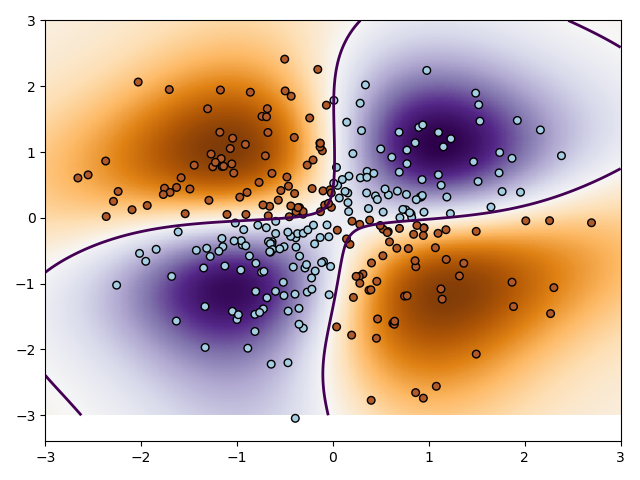## （三）SVM-人脸识别

http://www.hongweipeng.com/index.php/archives/1406/

## （四）make_blobs实验

https://www.jianshu.com/p/f7d2ed8aac54

# 六、拉格朗日乘子法

https://blog.csdn.net/on2way/article/details/47729419

1. 有拉格朗日乘法的地方，必然是一个组合优化问题。
2. 凸的就是开口朝一个方向（向上或向下）,拉格朗日法是一定适合于凸问题的，不一定适合于其他问题。
3. 有了约束(即等式 f(x)=2x**2+3y**2+7z**2，其中约束条件为2x+y=3,x+3z=9)不能直接求导，那么我们把这个约束乘一个系数加到目标函数中去，这样就相当于既考虑了原目标函数，也考虑了约束条件，即f(x)=2x**2+3y**2+7z**2+a(2x+y-3)+b(x+3z-9),分别对x\y\z求导等于零，带入约束条件，得到a\b;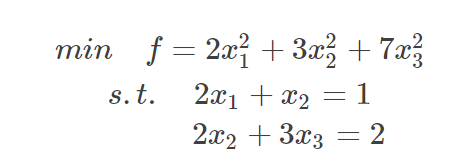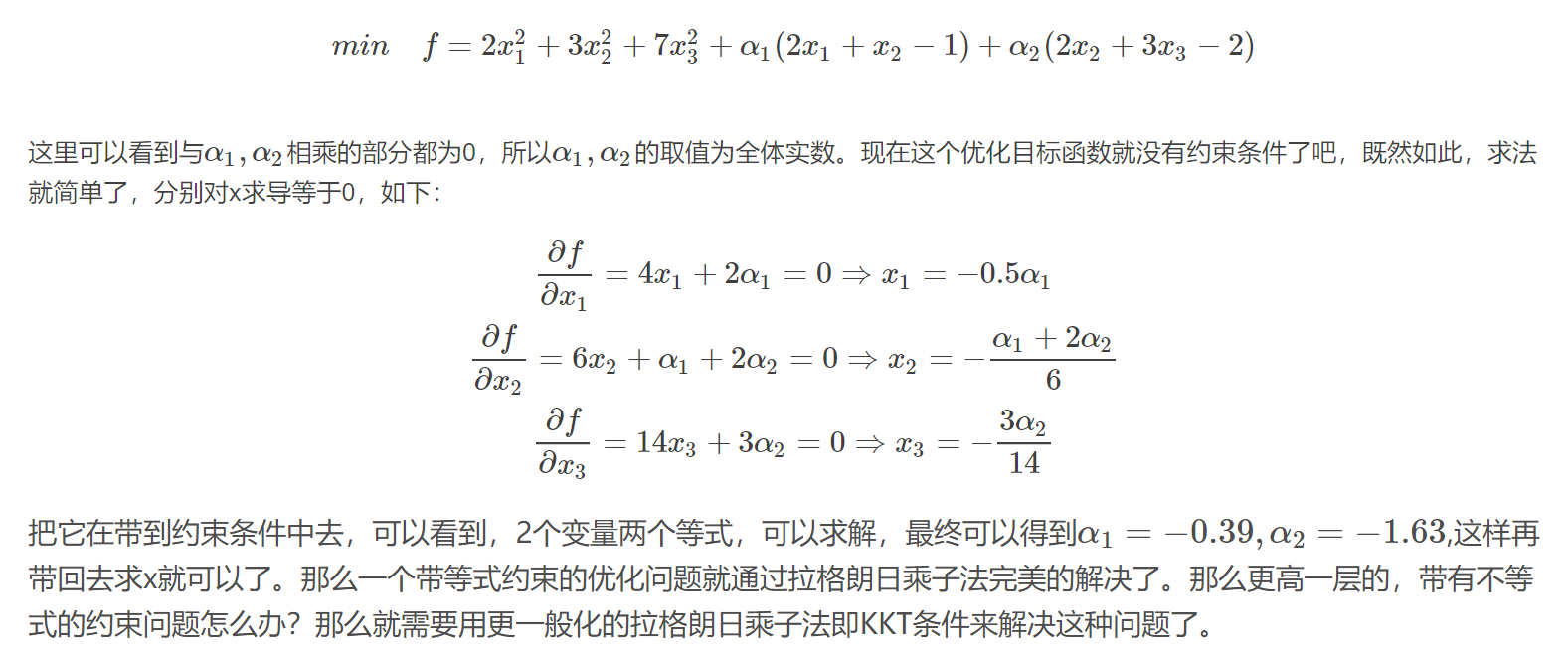# 七、KKT

1. 带有不等式的约束问题怎么办？就需要用更一般化的拉格朗日乘法即KKT条件来解决这种问题了。
2. KKT条件是解决最优化问题的时用到的一种方法,求其在指定作用域上的全局最小值。https://blog.csdn.net/on2way/article/details/47729419
3. 任何原始问题约束条件无非最多3种，等式约束，大于号约束，小于号约束，而这三种最终通过将约束方程化简化为两类：约束方程等于0和约束方程小于0。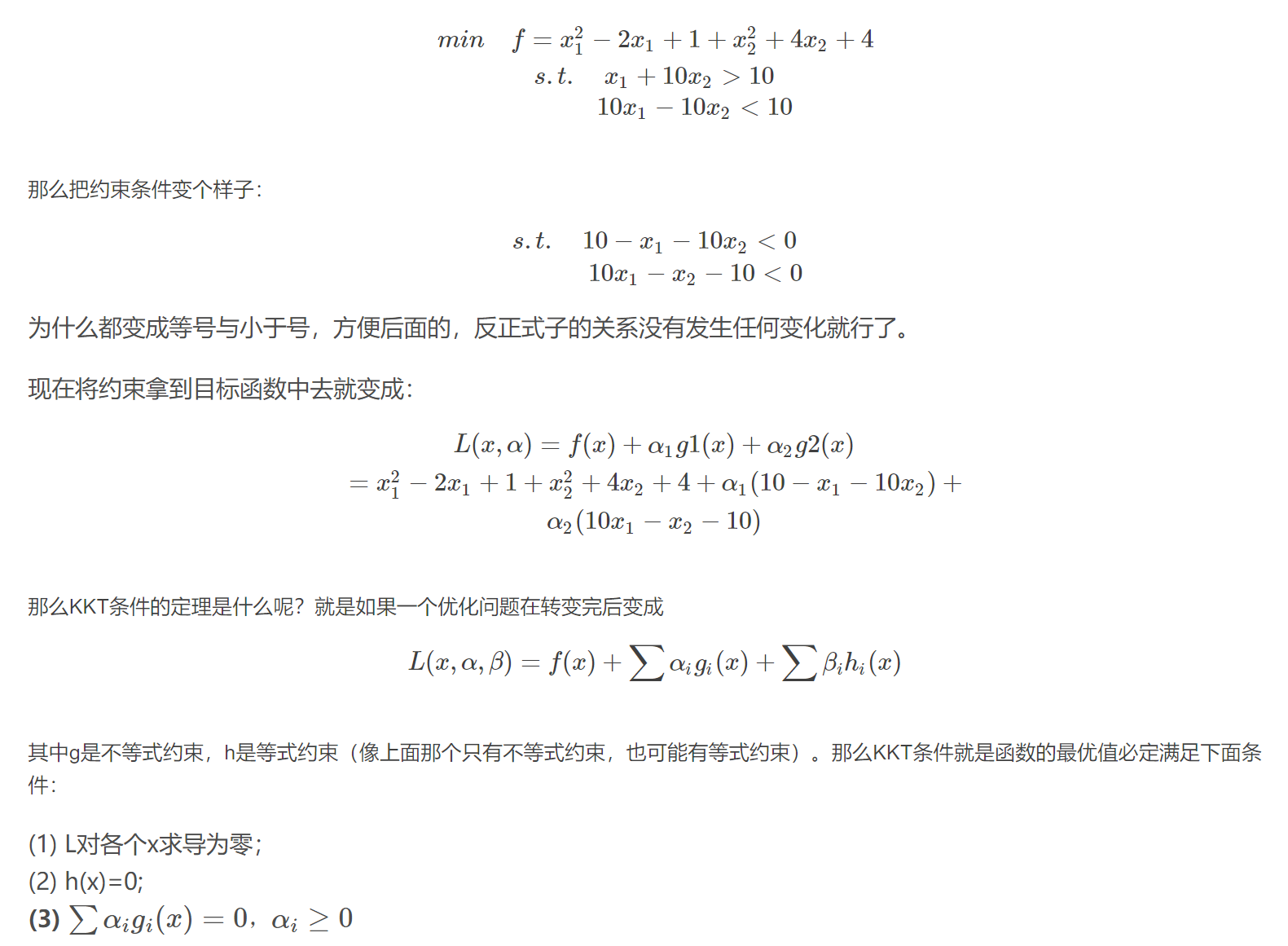# 十、总结

优点

• 可以有效的分离高维度的数据
• 更节省内存空间，因为只需要用向量来创建最佳超平面
• 是针对于可分离数据集的最佳方法

缺点

• 当数据量级较大的时候，分类效果不好
• 针对于不可分类的数据集，效果有些不太好
posted @ 2019-07-10 21:55  小婷儿  阅读(3103)  评论(0编辑  收藏  举报
levels of contents